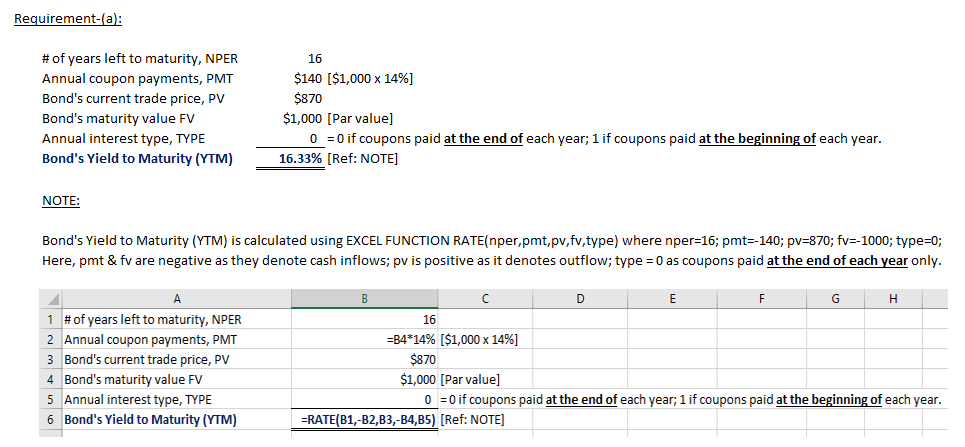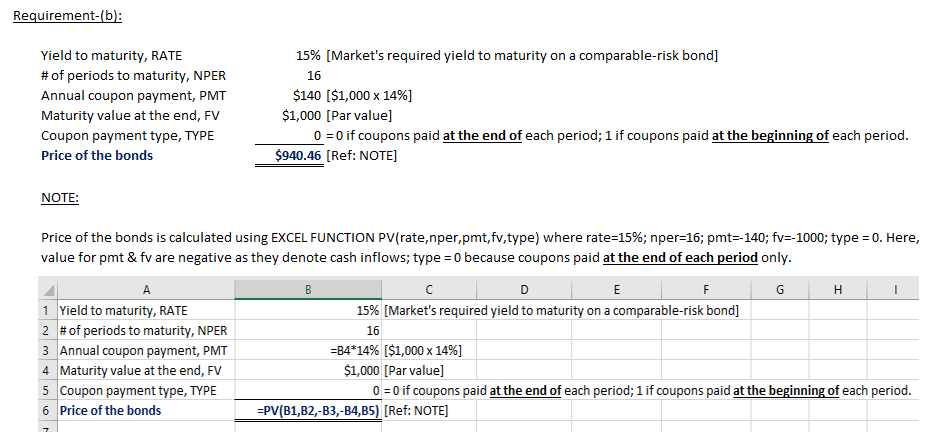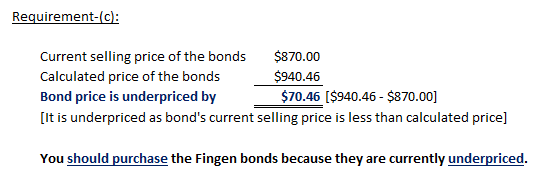In: Finance

# ​(Bond valuation) ​Fingen's 16​-year, $1,000 par value bonds pay 14 percent interest annually. The market price... 1. ​(Bond valuation) ​Fingen's 16​-year,$1,000 par value bonds pay 14 percent interest annually. The market price of the bonds is $870 and the​ market's required yield to maturity on a​ comparable-risk bond is 15 percent. a. Compute the​ bond's yield to maturity. b. Determine the value of the bond to​ you, given your required rate of return. c. Should you purchase the​ bond? a. What is your yield to maturity on the Fingen bonds given the market price of the​ bonds? _______ % (Round to two decimal​ places.) --> You should not OR should not purchase the Fingen bonds because they are currently overpriced OR underpriced. ## Solutions ##### Expert Solution## Related Solutions ##### (Bond valuation)?Fingen's 14?-year, ?$1,000 par value bonds pay 13 percent interest annually. The market price of...
(Bond valuation)?Fingen's 14?-year, ?$1,000 par value bonds pay 13 percent interest annually. The market price of the bonds is ?$870 and the? market's required yield to maturity on a? comparable-risk bond is 14 percent. a. Compute the? bond's yield to maturity. b. Determine the value of the bond to? you, given your required rate of return. c. Should you purchase the? bond? a. What is your yield to maturity on the Fingen bonds given the market price of the? bonds?...
##### ​Fingen's 12​-year, ​$1,000 par value bonds pay 15 percent interest annually. The market price of the... ​Fingen's 12​-year, ​$1,000 par value bonds pay 15 percent interest annually. The market price of the bonds is ​$880 and the​ market's required yield to maturity on a​ comparable-risk bond is 16 percent. a. Compute the​ bond's yield to maturity. b. Determine the value of the bond to​ you, given your required rate of return. c. Should you purchase the​ bond? ##### ​Fingen's 13​-year, ​$1,000 par value bonds pay 9 percent interest annually. The market price of the...
​Fingen's 13​-year, ​$1,000 par value bonds pay 9 percent interest annually. The market price of the bonds is ​$850 and the​ market's required yield to maturity on a​ comparable-risk bond is 10 percent. a. Compute the​ bond's yield to maturity. b. Determine the value of the bond to​ you, given your required rate of return. c. Should you purchase the​ bond?
##### Fingen's 15​-year, ​$1,000 par value bonds pay 12 percent interest annually. The market price of the... Fingen's 15​-year, ​$1,000 par value bonds pay 12 percent interest annually. The market price of the bonds is ​$1,100 and the​ market's required yield to maturity on a​ comparable-risk bond is 9 percent. a. Compute the​ bond's yield to maturity. b. Determine the value of the bond to​ you, given your required rate of return. c. Should you purchase the​ bond? ##### Fingen's 15​-year, ​$1,000  par value bonds pay 11 percent interest annually. The market price of the bonds...
Fingen's 15​-year, ​$1,000 par value bonds pay 11 percent interest annually. The market price of the bonds is ​$920 and the​ market's required yield to maturity on a​ comparable-risk bond is 14 percent. a.  Compute the​ bond's yield to maturity. b.  Determine the value of the bond to​ you, given your required rate of return. c.  Should you purchase the​ bond?
##### 7) Part 1. (Bond valuation)Fingen's 19?-year, ?$1,000 par value bonds pay 13 percent interest annually. The... 7) Part 1. (Bond valuation)Fingen's 19?-year, ?$1,000 par value bonds pay 13 percent interest annually. The market price of the bonds is ?$1,100 and the? market's required yield to maturity on a? comparable-risk bond is 10 percent. a. Compute the? bond's yield to maturity. b. Determine the value of the bond to? you, given your required rate of return. c. Should you purchase the? bond? Part 2. (Yield to? maturity) Abner? Corporation's bonds mature in 19 years and pay 11... ##### (Bond valuation​ relationships)The 14​-year,$1,000 par value bonds of Waco Industries pay 11 percent interest annually....
(Bond valuation​ relationships)The 14​-year, $1,000 par value bonds of Waco Industries pay 11 percent interest annually. The market price of the bond is$1,115​, and the​ market's required yield to maturity on a​ comparable-risk bond is 8 percent. a.  Compute the​ bond's yield to maturity. b.  Determine the value of the bond to you given the​ market's required yield to maturity on a​ comparable-risk bond. c.  Should you purchase the​ bond? 1) What is your yield to maturity on the...
##### ?(Bond valuation?) National? Steel's 10-year, ?$1,000 par value bonds pay 8 percent interest annually. The market... ?(Bond valuation?) National? Steel's 10-year, ?$1,000 par value bonds pay 8 percent interest annually. The market price of the bonds is ?$1,200?, and your required rate of return is 7 percent. a. Compute the? bond's expected rate of return. b. Determine the value of the bond to? you, given your required rate of return. c. Should you purchase the? bond? ##### ?(Bond valuation?) National? Steel's 10-year, ?$1,000 par value bonds pay 8 percent interest annually. The market...
?(Bond valuation?) National? Steel's 10-year, ?$1,000 par value bonds pay 8 percent interest annually. The market price of the bonds is ?$1,200?, and your required rate of return is 7 percent. a. Compute the? bond's expected rate of return. b. Determine the value of the bond to? you, given your required rate of return. c. Should you purchase the? bond?